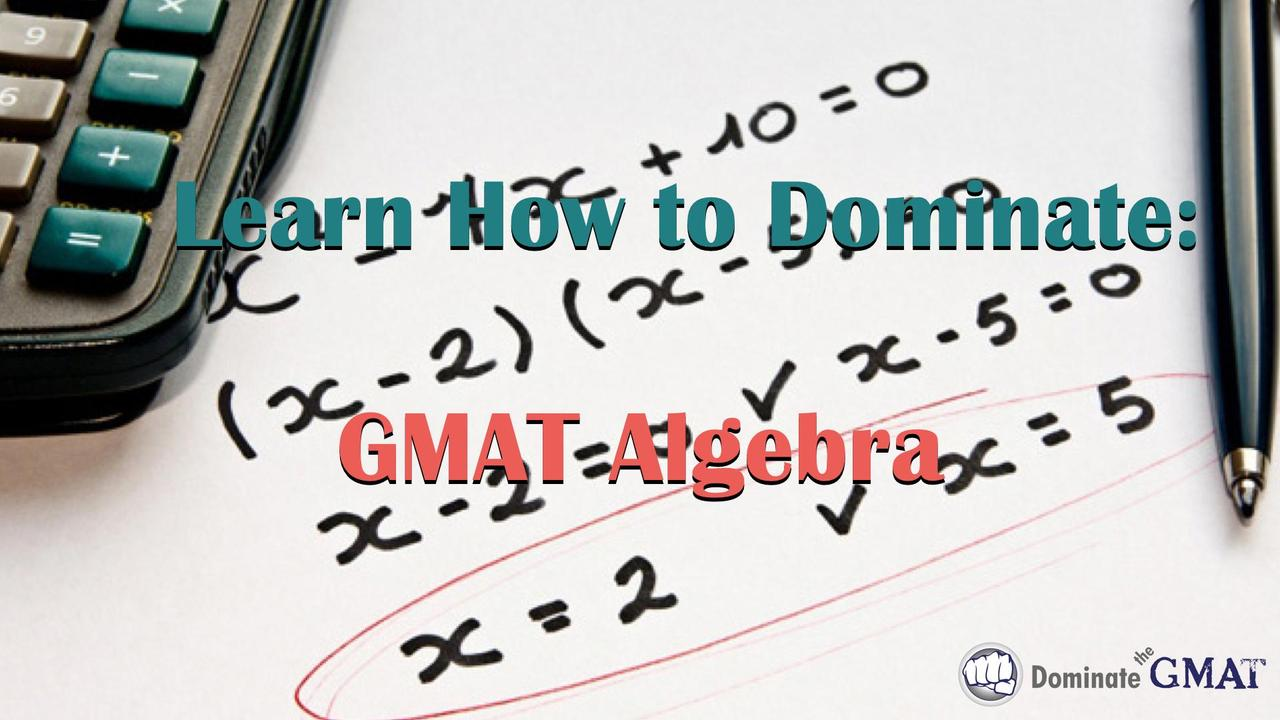# GMAT Algebra - Prep Course + Practice

We go to great lengths at Dominate Test Prep to help you avoid doing difficult algebra on the GMAT. There are useful non-standard math techniques, for example, that can help you make variables your friend and turn otherwise problematic word problems into run-of-the-mill math problems.

Sometimes, however, solving algebra problems on the GMAT is simply unavoidable.

In this course you will learn how to tackle several of the most commonly-tested “difficult” algebra concepts tested on the GMAT including quadratic equations, simultaneous equations, and inequalities. We'll also cover GMAT algebra questions that involve powers (exponents) and roots (radicals) as well as algebraic expressions that you may need to either simplify or manipulate in some way. But don’t worry — there won’t be a lot of long formulas to memorize (we still remember a song our 7th grade algebra teacher taught us to help us remember the Quadratic Formula, and don’t worry, we won’t be signing it to you here!); rather, we’ll focus on teaching you how the GMAT tests each of these topics and equip you with plenty of examples to illustrate everything you’ll need to master these GMAT algebra problem types and get more right answers on test day."I feel lucky to have come across Brett’s GMAT video tutorials. They broke down many of the barriers that I faced while trying to study for the GMAT on my own. It’s truly amazing to see how the quantitative problems on the GMAT can be methodically dissected and attacked with a strategic game-plan. Dominate the GMAT was nothing short of an excellent experience. The result? I dominated the GMAT and received an academic scholarship to my top-choice MBA program!" -- John Mason, USA

### Specifically, here's what this course covers:

• Simultaneous equations, with multiple approaches and strategies so that you can choose the method that works best for you;
• GMAT quadratic equations, with an emphasis on the three most commonly-tested quadratic equations on the GMAT;
• The FOIL Method, including how to factor quadratic equations using the “reverse FOIL” method;
• Figuring out how many roots a quadratic equation has for determining sufficiency on GMAT Data Sufficiency questions;
• The in's and out's of inequalities;
• GMAT exponents, including combining exponents by addition/subtraction and multiplication/division as well as a fool-proof strategy for avoiding the most common exponent traps found on the GMAT;
• Detailed review of roots and radicals;
• The most commonly-tested exponents and square roots on the GMAT;
• Simplifying algebraic expressions;
• Algebraic functions;
• Numerous application examples to fully illustrate all of the concepts taught in this course;
• And more!

### Additional features of the course include:

• Test your understanding with our 15-question practice worksheet (available as an online quiz or downloadable PDF) of GMAT algebra questions of varying difficulty levels;
• Detailed video answer explanations for each of those 15 sample problems;